# Getting Started with NNS: Clustering and Regression

require(NNS)
require(knitr)
require(rgl)
require(data.table)

# Clustering and Regression

Below are some examples demonstrating unsupervised learning with NNS clustering and nonlinear regression using the resulting clusters. As always, for a more thorough description and definition, please view the References.

## NNS Partitioning NNS.part

NNS.part is both a partitional and hierarchical clustering method. NNS iteratively partitions the joint distribution into partial moment quadrants, and then assigns a quadrant identification at each partition.

NNS.part returns a data.table of observations along with their final quadrant identification. It also returns the regression points, which are the quadrant means used in NNS.reg.

x = seq(-5, 5, .05); y = x ^ 3

for(i in 1 : 4){NNS.part(x, y, order = i, noise.reduction = "off", Voronoi = TRUE)}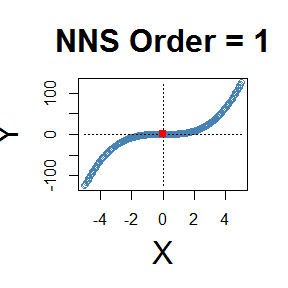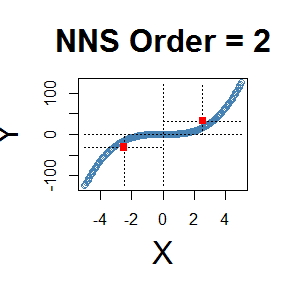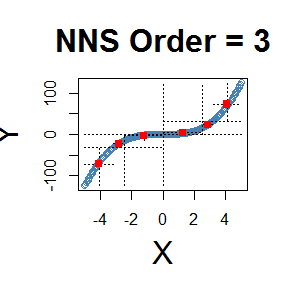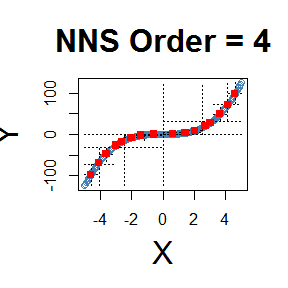### X-only Partitioning

NNS.part offers a partitioning based on $$x$$ values only, using the entire bandwidth in its regression point derivation, and shares the same limit condition as partitioning via both $$x$$ and $$y$$ values.

for(i in 1 : 4){NNS.part(x, y, order = i, type = "XONLY", Voronoi = TRUE)}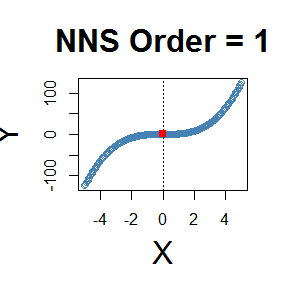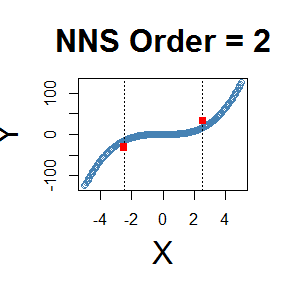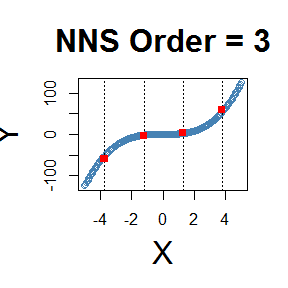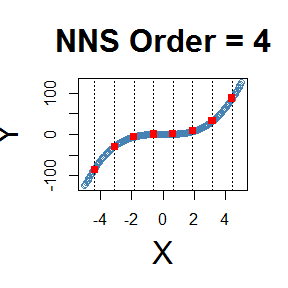## Clusters Used in Regression

The right column of plots shows the corresponding regression for the order of NNS partitioning.

for(i in 1 : 3){NNS.part(x, y, order = i, Voronoi = TRUE) ; NNS.reg(x, y, order = i)}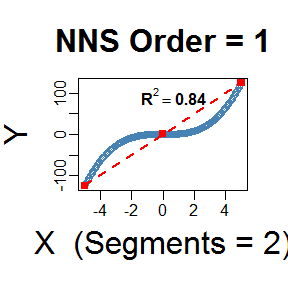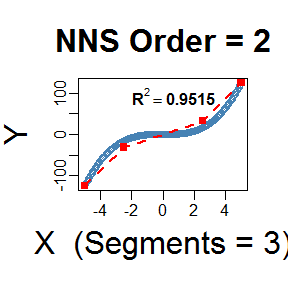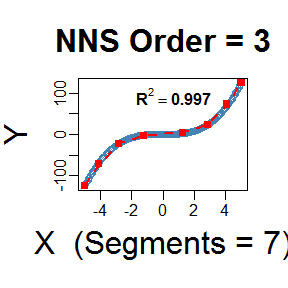## NNS Regression NNS.reg

NNS.reg can fit any $$f(x)$$, for both uni- and multivariate cases. NNS.reg returns a self-evident list of values provided below.

### Univariate:

NNS.reg(x, y, order = 4, noise.reduction = "off")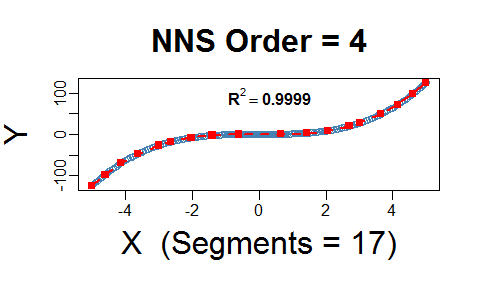## $R2 ##  0.9998899 ## ##$SE
##  0.7461974
##
## $Prediction.Accuracy ## NULL ## ##$equation
## NULL
##
## $x.star ## NULL ## ##$derivative
##     Coefficient X.Lower.Range X.Upper.Range
##  1:    67.09000        -5.000        -4.600
##  2:    58.87750        -4.600        -4.125
##  3:    43.66125        -4.125        -3.625
##  4:    34.04250        -3.625        -3.000
##  5:    24.00250        -3.000        -2.650
##  6:    15.96250        -2.650        -2.025
##  7:     9.48250        -2.025        -1.400
##  8:     2.92000        -1.400        -0.600
##  9:     0.78250        -0.600         0.650
## 10:     3.09250         0.650         1.425
## 11:     9.84250         1.425         2.050
## 12:    16.44250         2.050         2.700
## 13:    24.56250         2.700         3.025
## 14:    34.72250         3.025         3.650
## 15:    44.05000         3.650         4.150
## 16:    59.31250         4.150         4.600
## 17:    67.09000         4.600         5.000
##
## $Point ## NULL ## ##$Point.est
## NULL
##
## $regression.points ## x y ## 1: -5.000 -125.000000 ## 2: -4.600 -98.164000 ## 3: -4.125 -70.197187 ## 4: -3.625 -48.366563 ## 5: -3.000 -27.090000 ## 6: -2.650 -18.689125 ## 7: -2.025 -8.712562 ## 8: -1.400 -2.786000 ## 9: -0.600 -0.450000 ## 10: 0.650 0.528125 ## 11: 1.425 2.924813 ## 12: 2.050 9.076375 ## 13: 2.700 19.764000 ## 14: 3.025 27.746813 ## 15: 3.650 49.448375 ## 16: 4.150 71.473375 ## 17: 4.600 98.164000 ## 18: 5.000 125.000000 ## ##$Fitted
##          y.hat
##   1: -125.0000
##   2: -121.6455
##   3: -118.2910
##   4: -114.9365
##   5: -111.5820
##  ---
## 197:  111.5820
## 198:  114.9365
## 199:  118.2910
## 200:  121.6455
## 201:  125.0000
##

### Classification

For a classification problem, we simply set NNS.reg(x, y, type = "CLASS", ...).

NNS.reg(iris[ , 1 : 4], iris[ , 5], type = "CLASS", point.est = iris[141:150, 1 : 4], location = "topleft")$Point.est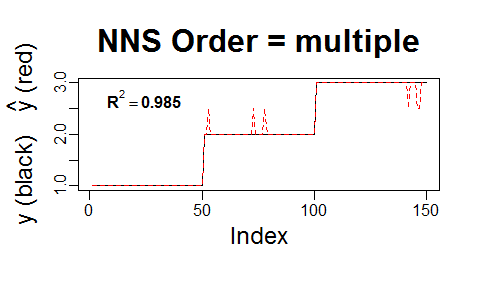##  3.0 2.5 3.0 3.0 3.0 2.5 2.5 3.0 3.0 3.0 ### NNS Dimension Reduction Regression NNS.reg also provides a dimension reduction regression by including a parameter NNS.reg(x, y, dim.red.method = "cor", ...). Reducing all regressors to a single dimension using the returned equation $equation.

NNS.reg(iris[ , 1 : 4], iris[ , 5], dim.red.method = "cor", location = "topleft")$equation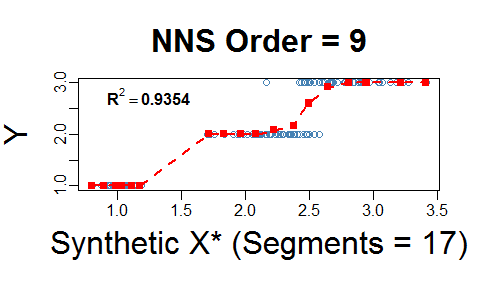## Variable Coefficient ## 1: Sepal.Length 0.7825612 ## 2: Sepal.Width -0.4266576 ## 3: Petal.Length 0.9490347 ## 4: Petal.Width 0.9565473 ## 5: DENOMINATOR 4.0000000 Thus, our model for this regression would be: $Species = \frac{0.7825612*Sepal.Length -0.4266576*Sepal.Width + 0.9490347*Petal.Length + 0.9565473*Petal.Width}{4}$ #### Threshold NNS.reg(x, y, dim.red.method = "cor", threshold = ...) offers a method of reducing regressors further by controlling the absolute value of required correlation. NNS.reg(iris[ , 1 : 4], iris[ , 5], dim.red.method = "cor", threshold = .75, location = "topleft")$equation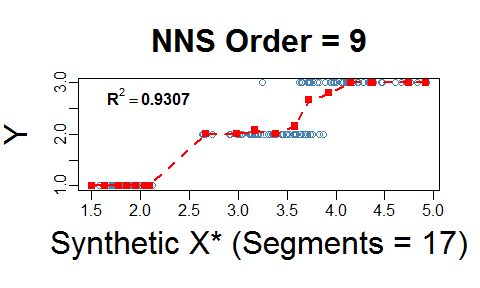##        Variable Coefficient
## 1: Sepal.Length   0.7825612
## 2:  Sepal.Width   0.0000000
## 3: Petal.Length   0.9490347
## 4:  Petal.Width   0.9565473
## 5:  DENOMINATOR   3.0000000

Thus, our model for this further reduced dimension regression would be: $Species = \frac{0.7825612*Sepal.Length -0*Sepal.Width + 0.9490347*Petal.Length + 0.9565473*Petal.Width}{3}$

and the point.est = (...) operates in the same manner as the full regression above, again called with $Point.est. NNS.reg(iris[ , 1 : 4], iris[ , 5], dim.red.method = "cor", threshold = .75, point.est = iris[1 : 10, 1 : 4], location = "topleft")$Point.est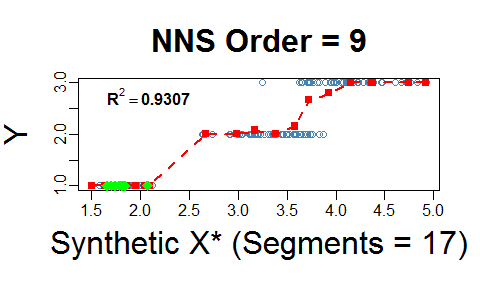##   1 1 1 1 1 1 1 1 1 1